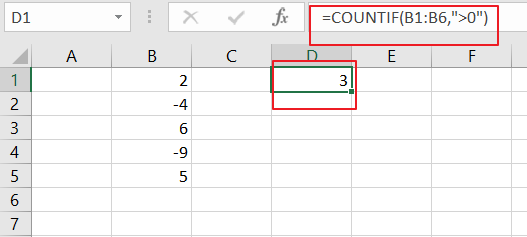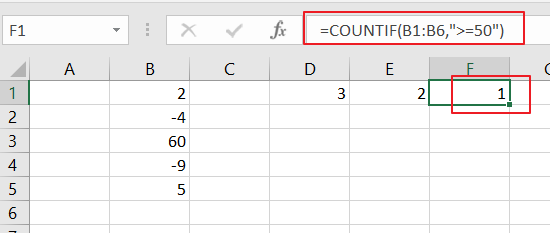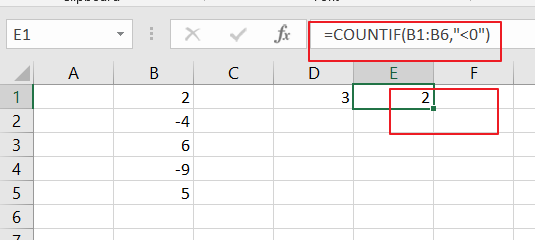# How to Count Cells that Contain Positive or Negative numbers in Excel

This post will guide you how to count the number of cells that only positive numbers within a range of cells using a formula in Excel 2013/2016 or Excel office 365.How do I count the number of cells that contain negative values using a simple formula in Excel. The below steps will show you how to use the COUNTIF function to count the number of cells that meet a certain criteria.

## Count Number of Cells that Contain Positive Numbers

Assuming that you have a data list in the range of cells B1:B6, and you want count the number of cells containing only positive numbers, and write the result in cell D1.You can use the below formula based on the COUNTIF function.

=COUNTIF(B1:B6,”>0″)LET’S SEE THAT HOW THIS FORMULA WORKS:

The COUNTIF function can be used to count the number of cells that match a single condition or criteria. And in this case, you need to provide a criteria as “`>0`”, which is evaluated as “values that need to grater than zero”. Then the total count of all cells in the range B1:B6 that meet the above criteria is returned.

You can also adjust this formula to count cell based on other criteria, such as, you wish to count cells with a value that greater than or equal to 50, and you can use the following formula:

=COUNTIF(B1:B6,”>=50)## Count Number of Cells that Contain Positive Numbers

If you wish to count number of cells that containing negative numbers within a range of cells B1:B6, and you can use the below formula based on the COUNTIF function. And you can provide a criteria “`<0`”, which is evaluated the values less than zero.

=COUNTIF(B1:B6,”<0”)### Related Functions

• Excel COUNTIF function
The Excel COUNTIF function will count the number of cells in a range that meet a given criteria. This function can be used to count the different kinds of cells with number, date, text values, blank, non-blanks, or containing specific characters.etc.= COUNTIF (range, criteria)…